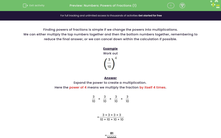# Numbers: Powers of Fractions (1)

In this worksheet, students work out powers of fractions.Key stage:  KS 3

Curriculum topic:   Number

Curriculum subtopic:   Use Four Operations for All Numbers

Difficulty level:#### Worksheet Overview

Finding powers of fractions is simple if we change the powers into multiplications.

We can either multiply the top numbers together and then the bottom numbers together, remembering to reduce the final answer, or we can cancel down within the calculation if possible.

Example

Work outExpand the power to create a multiplication.

Here the power of 4 means we multiply the fraction by itself 4 times.

 3 × 3 × 3 × 3 10 10 10 10

 = 3 × 3 × 3 × 3 10 × 10 × 10 × 10

OR

 = 34 104

 = 81 10000

### What is EdPlace?

We're your National Curriculum aligned online education content provider helping each child succeed in English, maths and science from year 1 to GCSE. With an EdPlace account you’ll be able to track and measure progress, helping each child achieve their best. We build confidence and attainment by personalising each child’s learning at a level that suits them.

Get started